# Oberbeck-Boussinesq equations

(diff) ← Older revision | Latest revision (diff) | Newer revision → (diff)

Equations giving an approximate description of the thermo-mechanical response of linearly viscous fluids (Navier–Stokes fluids or Newtonian fluids) that can only sustain volume-preserving motions (isochoric motions) in isothermal processes, but can undergo motions that are not volume-preserving during non-isothermal processes. Such a restriction on the response of the fluid requires that the determinant of the deformation gradientbe a function of the temperature, i.e.,(a1)

An approximation of the balance of mass, momentum and energy within the context of the above constraint was first discussed by A. Oberbeck [a1] and later by J. Boussinesq [a2]. Such an approximate system has relevance to a plethora of problems in astrophysics, geophysics and oceanography.

Numerous attempts have been made to provide a rigorous justification for the Oberbeck–Boussinesq equations, the details of which can be found in [a3].

While plausible arguments based on physical grounds are advanced to derive the Oberbeck–Boussinesq equations (see [a4]), namely that the effects of the variations in the density with respect to the temperature are more significant in the buoyancy forces than in the inertial effects, no compelling evidence or a rigorous mathematical basis is available.

The constraint (a1) implies thatwhere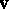is the velocity field,the temperature, andthe coefficient of thermal expansion.

Thus, while in an incompressible fluid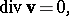(a2)

this is not necessarily so for fluids obeying (a1). However, in the Oberbeck–Boussinesq equations the constraint (a2) holds to within the order of approximation.

Letdenote the acceleration due to gravity, letbe a typical length scale (usually the thickness of the layer of the fluid),a representative density,the viscosity, and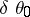a characteristic temperature difference. Assume thatis a constant. On introducing a characteristic velocityand characteristic timefor the problem through (see [a4])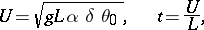one can set the relevant non-dimensional parameters to be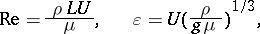where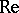is called the Reynolds number.

Assuming that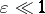andis a constant, and expressing the (non-dimensional) velocity, the temperatureand the pressurethroughand substituting the above into the governing equations for mass, momentum and energy balance for a Navier–Stokes fluid leads to an hierarchy of equations at different orders of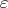. Let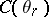denote the specific heat at some reference temperature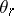and set,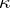being the thermal conductivity. Let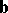denote the field of the body forces. Then the equations at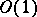read(a3)

Notice that the above set of equations is not adequate to determine the variables. When the equations for the balance of linear momentum at,and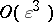are appended to (a3), i.e.,(a4)

one obtains a determinate system of equations (a3)–(a4), referred to as the Oberbeck–Boussinesq equations. Thus, the Oberbeck–Boussinesq equations do not follow from retaining the perturbances of the same order in. This perturbation procedure, discussed in [a3] in detail, also provides the corrections to the Oberbeck–Boussinesq equations at higher order of.

A similar heuristic approach has been developed for the thermo-mechanical response of non-Newtonian fluids [a5].

Also, in the case of the thermo-mechanical response of solids, the constraint (a1) seems to be applicable. However, the counterparts to the Oberbeck–Boussinesq equations have not yet been established as there is no single model that enjoys the kind of widespread use as the Navier–Stokes equations. From the point of view of mathematical analysis, the Oberbeck–Boussinesq equations are an example of a coupled non-linear system that retain the salient features of the Navier–Stokes equations when temperature effects are included. See [a6] or [a7] for details.

How to Cite This Entry:
Oberbeck-Boussinesq equations. Encyclopedia of Mathematics. URL: http://encyclopediaofmath.org/index.php?title=Oberbeck-Boussinesq_equations&oldid=14912
This article was adapted from an original article by Josef MÃ¡lekK.R. Rajagopal (originator), which appeared in Encyclopedia of Mathematics - ISBN 1402006098. See original article